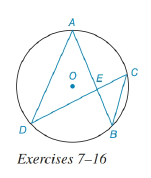Chapter 6.3, Problem 9EElementary Geometry For College St...

7th Edition
Alexander + 2 others
ISBN: 9781337614085

Solutions

Chapter
SectionElementary Geometry For College St...

7th Edition
Alexander + 2 others
ISBN: 9781337614085
Textbook Problem

In the figure for Exercises 7 to 16, O is the center of the circle. See Theorem 6.3.5.Given: A E = 8 , E B = 6 , D C = 16 Find: DE and EC.To determine

To find:

To find DE and EC.

Explanation

Given that AE=8,EB=6,DC=16.

The diagrammatic representation is given below,

Theorem:

If two chords intersect within a circle, then the product of the lengths of the segments (parts) of one chord is equal to the product of the lengths of the segments of the other chord.

By using the theorem to get the following,

AEEB=DEEC...(1)

Also, know that DC=DE+EC...(2)

Substitute the values AE=8,EB=6,DC=16 in equation (1) and (2) to get the following,

AEEB=DEEC86=DEECDEEC=48DE=48EC...(3)

DE+EC=16...(4)

Substitute equation (3) in equation (4) to get the following,

DE+EC=1648EC+EC=1648+EC2=16ECEC216EC+48=0

Solve the above quadratic equation to get the following,

Still sussing out bartleby?

Check out a sample textbook solution.

See a sample solution

The Solution to Your Study Problems

Bartleby provides explanations to thousands of textbook problems written by our experts, many with advanced degrees!

Get Started

Solve the equations in Exercises 126. (x21)2(x+2)3(x21)3(x+2)2=0

Finite Mathematics and Applied Calculus (MindTap Course List)

Find the limit or show that it does not exist. limxx+3x24x1

Single Variable Calculus: Early Transcendentals, Volume I

1. Write the simplex matrix to maximize subject to the constraints

Mathematical Applications for the Management, Life, and Social Sciences

True or False: is a convergent series.

Study Guide for Stewart's Multivariable Calculus, 8th

The graph of x = 2 + 3t, y = 4 − t is a: circle ellipse line parabola

Study Guide for Stewart's Single Variable Calculus: Early Transcendentals, 8th